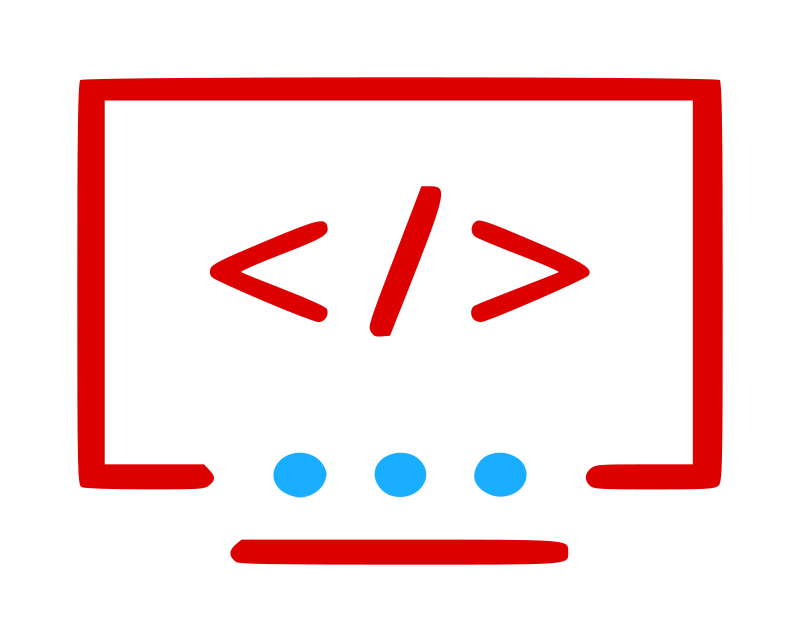# Mastering PHP 8.2: Disjunctive Normal Form Types‍OpenClipart Image by ben

‍As a web developer, keeping up with the latest technology is essential to stay relevant and competitive in the industry. With the release of PHP 8.2, there are new features and improvements that can help you elevate your web development skills. In this article we cover a new feature of PHP 8.2 called disjunctive normal form types and provide you with short code examples to aid your understanding.

## Disjunctive Normal Form (DNF)

Disjunctive Normal Form (DNF) is a canonical normal form of a logical formula consisting of a disjunction of conjunctions, or simply an OR of ANDs. It represents a logical formula as the alternation of one or more conjunctions of one or more literals. In PHP 8.2, you can use DNF to simplify complex boolean expressions, making your code more readable and maintainable. Before showing you an example, you first need to understand two other types, introduced in slightly earlier versions of PHP: union and intersection types.

## Union Types

In this example, the first argument \$data accepts either an array or a string as a data type. This is referred to as a union type because it's a union of `array` and `string`. Note that the OR symbol ("`|`") is used to represent the union. Please note that you're not limited to just two types. You can add others if appropriate.

```function get_row(array|string \$data, bool \$td = TRUE) {     \$tag = (\$td)             ? function (\$item) { return '' . \$item . ''; }             : function (\$item) { return '' . \$item . ''; };     \$html = '';     if (is_string(\$data)) {         \$data = str_getcsv(\$data);     } elseif (!is_array(\$data)) {         \$data = [];     }     foreach (\$data as \$item) \$html .= \$tag(\$item);     \$html .= '' . PHP_EOL;     return \$html; }```

See Code

## Intersection Types

The intersection type was introduced in PHP 8.1 and is mainly useful in OOP. Whereas union types allows you to pass either of the types specified, the intersection type means that the object you pass need to match all of the intersecting types. In this example, the function `add_and_get_avg()` requires an object that implements all of these interfaces: `ArrayAccess,` `Countable` and `Traversable.` Note that the AND symbol ("`&`") is used to represent an intersection of types.

```function add_and_get_avg(ArrayAccess&Countable&Traversable \$obj, float \$value) : float {     // ArrayAccess allows object to act as array     \$obj[] = \$value;     \$sum = 0;     // Traversable allows object to be used in a foreach loop     foreach (\$obj as \$val) \$sum += \$val;     // Countable lets you use the count() function on the object     return \$sum / count(\$obj); }```

`See Code`

## Disjunctive Normal Form

OK, now we're ready to talk about DNF. In the code example shown in the section discussing intersection types, you've no doubt recognized that a simple array can easily handle the logic inside the function. However, prior to PHP 8.2 you would need to remove the type hints entirely in order to allow objects that implement those three interfaces and also allow arrays! As of PHP 8.2, you can use parentheses to subgroup types and gang them together in whatever form is appropriate.

In this example, we can make a couple of simple modifications to the function signature to achieve the desired result:

```function add_and_get_avg((ArrayAccess&Countable&Traversable)|array &\$obj, float \$value) : float```

We placed paratheses around the intersection type, and placed the intersection type in union with `array`. Note that we also needed to add an ampersand ("`&`") in front of `\$obj` so that the array can be passed by reference. This has no effect on objects as they are already implicitly passed by reference.

In the runner code, this now works:

```\$arr = [1,2]; echo "Current Avg: " . add_and_get_avg(\$arr, 3) . "\n";    // 2 echo "Current Avg: " . add_and_get_avg(\$arr, 4) . "\n";    // 2.5 echo "Current Avg: " . add_and_get_avg(\$arr, 5) . "\n"; // 3```

See Code